### Another HeroHover over the thumbnail for a full-size version.

Author wedgie123 author:wedgie123 bitesized n-art rated 2006-01-31 2006-01-31 4 by 75 people. \$Another Hero#wedgie123#none#00000000000000000000000000000000000000000000000000000000000000000000000000000000000000000000000000000000000000000000000000000000000000000000000000000000000000000000000000000000000000000000000000000000000000000000000000000000000000000000000000000000000000000000000000000000000000000000000000000000000000000000000000000000000000000000000000000000000000000000000000000000000000000000000000000000000000000000000000000000000000000000000000000000000000000000000000000000000000000000000000000000000000000000000000000000000000000000000000000000000000000000000000000000000000000000000000000000000000000000000000000000000000000000000000000000000000000000000000000000000000000000000000000000000000000000000000000000000000000|5^517,54!5^523,55!5^524,55!5^525,55!5^558,53!3^233,352!3^231,368!3^230,383!3^229,396!3^228,410!3^231,426!3^236,443!3^244,469!3^240,456!3^252,484!3^248,478!3^265,508!3^259,499!3^255,491!3^276,518!3^270,513!3^295,537!3^286,529!3^279,522!3^323,559!3^315,553!3^308,546!3^302,542!3^354,574!3^344,573!3^337,569!3^330,565!3^361,576!3^373,571!3^372,575!3^381,575!3^392,571!3^401,564!3^414,553!3^425,542!3^432,529!3^439,515!3^445,501!3^456,484!3^472,467!3^483,449!3^496,426!3^505,401!3^514,378!3^520,362!3^211,345!3^205,362!3^200,380!3^196,398!3^188,419!3^178,435!3^166,455!3^154,476!3^138,493!3^125,505!3^112,517!3^123,528!3^172,572!3^163,563!3^152,556!3^143,548!3^130,538!3^104,525!3^90,535!3^75,544!3^58,551!3^50,560!3^42,569!3^147,484!3^131,501!3^114,515!3^162,466!3^173,445!3^183,425!3^193,408!3^331,551!3^324,547!3^313,539!3^306,532!3^298,526!3^292,520!3^286,514!3^274,502!3^267,495!3^280,509!3^230,421!3^240,431!3^244,439!3^249,448!3^250,456!3^253,464!3^255,472!3^259,481!3^231,432!3^237,449!3^245,459!3^263,489!3^374,566!3^365,568!3^356,566!3^349,563!3^339,560!3^331,556!3^358,572!3^348,569!3^339,563!3^330,557!3^321,550!3^311,545!3^302,539!3^294,532!3^313,525!3^300,509!3^288,496!3^276,484!3^264,462!3^259,446!3^252,432!3^246,419!3^235,416!3^236,404!3^236,389!3^215,350!3^223,350!3^220,355!3^220,363!3^220,370!3^218,377!3^218,383!3^217,391!3^217,398!3^210,398!3^209,389!3^209,379!3^209,377!3^209,369!3^210,361!3^210,354!3^212,367!3^200,377!3^200,389!3^203,371!3^210,354!3^200,391!3^214,387!3^214,382!3^214,373!3^214,368!3^216,361!3^221,351!3^215,399!3^216,402!3^216,409!3^216,412!3^206,421!3^207,416!3^207,407!3^207,402!3^207,397!3^205,401!3^199,408!3^195,415!3^191,421!3^199,414!3^211,401!3^204,416!3^209,425!3^202,430!3^194,430!3^187,443!3^200,436!3^212,435!3^196,445!3^184,451!3^173,461!3^205,446!3^215,423!3^195,453!3^183,463!3^170,472!3^191,433!3^367,500!3^381,506!3^390,508!3^401,511!3^416,510!3^427,504!3^441,495!3^401,455!3^423,459!3^448,466!3^453,475!3^391,456!10^313,551!10^308,548!10^304,544!10^297,539!10^293,535!10^284,529!10^281,526!10^276,519!10^273,515!10^268,510!10^265,504!10^261,500!10^258,494!10^255,490!10^253,486!10^316,555!10^321,559!10^248,479!10^246,473!10^241,465!3^236,468!3^236,479!3^236,492!3^238,507!3^239,522!3^242,536!3^260,520!3^255,513!3^248,504!3^245,497!3^241,488!3^251,525!3^245,516!3^242,509!3^237,498!3^237,492!3^237,484!3^236,476!3^235,461!3^241,487!3^243,494!3^248,501!3^251,511!3^246,516!3^243,505!3^240,499!3^240,508!3^240,518!3^243,529!3^246,536!3^248,562!3^243,549!3^243,543!3^252,531!3^251,542!3^250,552!3^267,530!3^261,536!3^261,548!3^255,562!3^264,566!3^267,558!3^271,540!3^279,540!3^278,552!3^272,569!3^280,561!3^287,546!3^300,553!3^290,559!3^285,567!3^296,570!3^304,563!3^308,572!3^311,567!3^323,573!3^277,572!3^317,574!3^265,575!3^250,574!3^260,525!3^236,470!3^236,481!3^237,500!3^232,518!3^231,504!3^232,511!3^232,492!3^232,476!3^232,457!3^238,527!3^233,525!3^234,537!3^236,557!3^255,515!3^250,506!3^241,491!3^241,481!3^242,500!3^234,504!3^234,490!3^234,476!3^221,440!3^224,449!3^224,465!3^224,482!3^225,500!3^281,538!3^274,530!3^268,524!3^263,513!3^260,506!3^252,496!3^249,488!3^276,525!3^263,531!3^258,539!3^255,554!3^292,544!3^282,535!3^274,529!3^267,521!3^259,511!3^258,503!3^223,556!3^223,542!3^195,567!3^208,559!3^215,567!3^234,568!3^225,574!3^246,574!3^206,573!3^122,518!3^141,505!3^163,481!3^148,495!3^156,486!3^133,509!3^129,519!3^138,531!3^146,517!3^156,506!3^169,484!3^182,466!3^156,493!3^140,522!11^8,495,114,531!11^9,518,103,534!11^7,518,96,540!11^10,523,107,543!11^7,520,117,539!11^7,547,87,546!11^6,530,96,550!11^2,525,105,551!11^4,508,116,548!11^4,524,122,546!11^13,525,52,572!11^7,530,58,566!11^8,535,60,562!11^6,532,66,557!11^5,525,76,554!11^4,523,63,574!11^4,529,70,568!11^5,527,72,561!11^1,526,82,559!11^7,521,80,569!11^9,538,73,575!11^2,525,90,557!11^7,521,132,548!11^4,520,90,564!11^7,523,98,559!11^3,515,124,555!11^5,520,109,558!11^11,534,88,571!11^8,519,100,566!11^6,518,111,566!11^15,513,123,563!11^6,518,134,556!11^4,527,136,565!11^2,515,141,561!11^9,525,145,570!11^6,532,152,566!11^7,541,158,573!11^6,541,98,574!11^7,537,107,574!11^5,518,126,569!11^7,524,136,573!11^7,528,140,574!11^5,520,150,575!11^1,521,123,573!11^5,506,114,572!3^209,459!3^209,481!3^210,511!3^207,543!3^218,523!3^220,513!3^207,495!3^210,470!3^214,446!3^218,491!3^201,529!3^197,507!3^195,483!3^195,463!3^191,551!3^177,562!3^229,570!3^230,560!3^234,551!3^235,539!3^237,524!3^217,573!3^222,557!3^228,541!3^235,530!3^238,570!3^247,563!3^250,524!3^248,519!3^248,502!3^244,492!3^237,478!3^237,465!3^237,462!10^367,570!10^359,571!10^353,569!10^345,567!10^338,566!10^329,562!10^324,559!10^389,569!10^384,570!10^378,570!10^369,570!10^363,570!10^383,572!10^375,574!10^364,573!10^392,570!10^312,552!10^304,545!10^295,539!3^280,542!3^286,545!3^292,553!3^297,558!3^313,566!3^329,574!3^305,562!3^296,552!3^274,546!3^303,554!3^290,540!3^272,530!3^395,560!3^383,560!3^375,560!3^363,557!3^353,555!3^342,549!3^330,541!3^404,555!3^395,520!3^386,540!3^396,537!3^368,531!3^390,517!3^373,512!3^382,532!3^382,544!3^379,554!3^389,528!3^420,535!3^393,546!3^376,529!3^376,542!3^367,564!3^362,546!3^351,543!3^350,532!3^326,530!3^340,539!3^346,549!3^360,558!3^374,558!3^334,551!3^363,545!3^347,562!3^342,559!10^229,428!10^230,436!10^237,446!10^239,453!10^242,460!10^238,445!10^233,440!10^231,435!3^221,427!3^221,415!3^232,457!3^229,446!3^234,454!12^367,477!12^448,482!12^448,484!12^415,500!12^407,501!12^395,500!12^401,501!12^372,470!12^367,470!12^378,467!12^386,467!12^397,465!12^407,465!12^415,465!12^421,470!12^425,471!12^430,469!12^443,478!12^440,472!12^437,470!3^371,471!3^379,471!3^389,470!3^380,461!3^372,461!3^364,468!3^371,498!3^407,507!3^399,506!3^387,502!3^378,497!3^435,499!3^417,503!3^439,509!12^365,475!3^150,540!3^155,521!3^167,495!12^392,467!12^404,464!12^443,486!3^394,378!3^389,385!3^386,394!3^387,404!3^359,473!12^421,501!12^428,498!12^435,495!12^441,489!10^412,482!10^427,479!10^420,481!10^416,483!10^408,481!10^403,477!10^396,476!10^389,474!10^382,473!10^375,473!10^399,476!10^393,474!10^383,472!10^375,476!10^367,476!10^432,479!10^437,479!10^439,482!10^422,481!3^413,472!3^405,470!3^416,473!3^410,486!3^405,484!3^398,479!3^417,483!3^431,486!3^423,487!3^416,490!3^397,497!3^390,495!3^382,491!3^374,487!3^419,549!3^397,568!3^403,565!3^410,559!3^427,536!3^450,492!3^454,488!3^462,478!3^466,473!3^487,439!3^498,416!3^504,408!3^490,434!3^509,393!3^512,386!3^477,461!3^445,507!3^450,497!3^449,474!3^440,464!3^431,464!3^426,464!3^412,461!3^385,461!3^449,436!3^476,414!3^470,417!3^459,418!3^463,418!3^454,426!3^451,419!3^450,420!3^414,543!3^405,546!3^384,557!3^345,519!3^330,517!3^329,503!3^264,476!3^292,511!3^277,497!3^247,438!3^236,425!3^307,517!3^320,520!9^397,416,0,1,0,19,0,-1,0!9^394,410,0,1,0,17,0,-1,0!9^392,400,0,1,0,15,0,-1,0!9^396,387,0,1,0,13,0,-1,0!9^404,379,0,1,0,14,0,-1,0!9^401,383,0,1,0,15,0,-1,0!9^392,394,0,1,0,16,0,-1,0!9^394,406,0,1,0,18,0,-1,0!3^322,552!3^324,545!3^316,543!3^466,329!3^467,339!3^467,350!3^467,362!3^467,368!3^469,381!3^473,391!3^475,400!3^467,407!3^464,396!3^455,409!9^461,426,0,1,0,16,0,-1,0!9^463,423,0,1,0,17,0,-1,0!9^469,423,0,1,0,16,0,-1,0!9^473,422,0,1,0,15,0,-1,0!9^475,420,0,1,0,15,0,-1,0!10^414,415!10^411,417!10^421,415!10^429,415!10^425,417!10^419,417!10^432,417!10^432,424!10^426,422!10^418,421!3^440,427!3^440,423!3^436,415!3^432,430!3^422,428!3^404,422!10^402,417!10^398,414!3^415,428!3^463,468!3^412,458!3^450,311!3^440,321!3^428,331!3^376,346!3^348,317!3^340,308!3^333,299!3^314,279!3^464,425!3^501,416!9^471,332,0,1,32,13,0,0,0!9^471,337,0,1,32,14,0,0,0!9^470,342,0,1,32,15,0,0,0!9^470,350,0,1,32,17,0,0,0!9^470,357,0,1,32,19,0,0,0!9^470,364,0,1,32,21,0,0,0!9^469,369,0,1,32,23,0,0,0!9^472,375,0,1,32,16,0,0,0!9^473,383,0,1,32,17,0,0,0!9^475,390,0,1,32,18,0,0,0!9^478,398,0,1,32,18,0,0,0!9^478,405,0,1,32,18,0,0,0!9^477,413,0,1,32,20,0,0,0!9^475,420,0,1,32,22,0,0,0!9^411,308,0,1,32,12,0,0,0!9^409,300,0,1,32,10,0,0,0!9^405,293,0,1,32,9,0,0,0!9^394,284,0,1,32,8,0,0,0!9^385,278,0,1,32,7,0,0,0!9^375,275,0,1,32,6,0,0,0!9^367,275,0,1,32,5,0,0,0!9^351,275,0,1,32,8,0,0,0!9^343,280,0,1,32,7,0,0,0!9^408,310,0,1,32,11,0,0,0!9^404,310,0,1,32,11,0,0,0!9^396,308,0,1,32,9,0,0,0!9^386,308,0,1,32,9,0,0,0!9^367,303,0,1,32,9,0,0,0!9^358,296,0,1,32,9,0,0,0!9^351,290,0,1,32,9,0,0,0!9^358,281,0,1,32,10,0,0,0!9^358,292,0,1,32,10,0,0,0!9^385,283,0,1,32,9,0,0,0!9^383,290,0,1,32,9,0,0,0!9^380,294,0,1,32,9,0,0,0!9^373,298,0,1,32,10,0,0,0!10^374,288!10^378,286!10^377,292!10^373,295!10^370,296!10^367,296!10^364,293!10^364,290!10^363,288!10^361,288!10^358,290!10^359,285!10^369,285!10^366,283!10^368,282!10^370,282!10^373,283!10^374,284!10^374,284!10^376,286!10^377,287!10^378,287!10^380,286!10^373,292!10^366,294!10^364,294!10^362,295!10^361,290!10^363,282!10^362,282!10^364,282!10^365,282!10^370,280!10^372,280!10^375,280!10^368,278!9^398,288,0,1,32,9,0,0,0!9^347,285,0,1,32,7,0,0,0!9^347,278,0,1,32,6,0,0,0!9^355,275,0,1,32,9,0,0,0!9^361,275,0,1,32,8,0,0,0!9^358,273,0,1,32,8,0,0,0!9^365,273,0,1,32,9,0,0,0!9^370,274,0,1,32,9,0,0,0!9^379,308,0,1,32,11,0,0,0!9^371,306,0,1,32,12,0,0,0!9^398,310,0,1,32,12,0,0,0!9^389,283,0,1,32,11,0,0,0!9^410,304,0,1,32,12,0,0,0!9^380,277,0,1,32,10,0,0,0!9^374,276,0,1,32,9,0,0,0!3^419,332!3^429,321!3^439,312!3^440,309!3^445,301!3^439,292!3^432,297!3^423,303!3^430,306!3^427,286!3^442,313!3^428,325!3^428,314!3^435,305!3^437,303!3^435,297!3^428,288!3^403,346!3^365,343!3^353,334!3^338,318!3^326,308!3^378,334!3^370,323!3^379,324!3^403,322!3^415,316!3^406,320!3^396,321!3^388,319!3^379,316!3^370,316!3^359,310!3^351,303!3^345,298!3^341,292!3^338,288!3^361,320!3^352,313!3^384,324!3^425,298!3^422,298!3^420,295!3^416,288!3^411,280!3^407,274!3^401,271!3^417,288!3^412,285!3^404,277!3^400,274!3^392,268!3^333,283!3^329,276!3^334,271!3^342,267!3^349,262!3^356,262!3^367,261!3^374,264!3^383,267!3^391,269!3^387,269!3^378,264!3^366,262!3^361,262!3^351,262!3^347,265!3^336,269!3^336,272!3^333,275!3^346,256!3^312,271!3^406,315!3^394,315!3^384,314!3^363,310!3^351,301!10^428,277!10^425,274!10^421,270!10^377,254!10^433,274!10^427,270!10^426,269!10^422,267!10^419,264!10^412,260!10^408,258!10^404,256!10^401,255!10^398,253!10^394,251!10^389,249!10^386,248!10^380,247!10^375,246!10^377,253!10^381,253!10^383,253!10^386,253!10^392,253!10^398,254!10^403,254!10^411,256!10^415,259!10^433,268!10^431,266!10^428,262!10^426,260!10^422,259!10^419,257!10^416,254!10^413,253!10^409,251!10^405,250!10^401,249!10^395,249!10^394,249!10^389,249!10^382,249!10^371,248!10^371,244!10^363,247!10^355,246!10^339,246!10^348,242!10^355,242!10^366,242!10^333,243!10^451,280!10^440,279!10^437,279!10^440,271!10^445,275!10^447,284!3^450,288!3^456,287!3^456,276!3^451,272!3^438,266!3^435,263!3^427,257!3^322,252!3^328,252!3^336,250!3^340,250!3^352,247!3^311,250!3^311,250!3^317,248!3^318,247!3^327,244!3^329,244!3^334,244!3^344,246!3^344,246!3^344,244!3^311,257!3^313,291!3^299,272!3^299,265!3^301,261!3^322,264!3^413,327!3^398,331!3^385,331!3^382,343!3^399,341!3^412,338!3^422,317!3^423,326!3^409,342!3^393,348!3^434,308!3^332,292!3^314,282!3^321,279!3^325,294!3^329,285!3^326,284!3^333,298!3^338,301!3^317,274!3^327,269!3^333,265!3^366,252!3^349,252!3^334,254!3^289,241!3^291,233!3^305,202!3^300,215!3^292,223!3^288,228!3^283,244!3^277,251!3^266,258!3^261,261!3^251,265!3^239,316!3^216,332!3^204,328!3^193,321!3^213,336!3^185,280!3^185,268!3^187,255!3^187,236!3^194,222!3^202,216!3^211,232!3^212,221!3^189,248!3^220,242!3^215,235!3^212,227!3^218,244!3^222,258!3^223,266!3^226,281!3^228,290!3^224,275!3^223,253!3^197,199!3^202,152!3^222,106!3^257,65!3^544,67!3^544,77!3^567,84!3^589,101!3^602,182!3^591,165!3^576,156!3^583,181!3^588,202!3^590,232!3^583,248!3^567,260!3^555,271!3^538,273!3^544,258!3^547,244!3^547,225!3^547,202!3^544,187!3^537,175!3^466,146!3^407,162!3^410,146!3^380,144!3^364,160!3^357,174!3^355,184!3^285,198!3^245,258!3^254,237!3^262,223!3^272,206!3^245,250!3^239,269!3^239,276!3^298,185!3^306,172!3^330,154!3^431,317!3^436,331!3^390,358!3^402,353!3^375,356!3^454,300!3^399,367!3^293,281!3^295,259!3^227,337!3^472,312!3^468,310!3^463,310!3^472,322!3^467,318!3^478,309!3^472,302!3^464,300!3^461,300!3^464,268!3^480,269!3^491,276!3^499,283!3^498,289!3^489,290!3^480,296!3^467,293!3^474,286!3^489,284!3^477,274!3^472,272!3^464,282!3^448,260!3^468,268!3^461,263!3^439,259!3^449,264!3^422,306!3^415,304!3^413,295!3^408,289!3^397,280!3^384,272!3^369,267!3^350,267!3^338,269!3^341,272!3^332,280!9^478,317,0,1,32,9,0,0,0!9^481,332,0,1,32,11,0,0,0!9^502,341,0,1,32,11,0,0,0!9^480,313,0,1,32,10,0,0,0!9^493,311,0,1,32,10,0,0,0!9^512,318,0,1,32,11,0,0,0!10^495,323!10^499,318!10^496,315!10^493,315!10^489,317!10^485,317!10^482,319!10^481,324!10^484,328!10^485,330!10^488,329!10^493,329!10^498,325!10^499,322!10^493,324!10^492,324!10^491,324!10^491,324!10^486,323!10^486,321!10^487,319!10^489,318!10^494,327!10^496,327!10^494,328!10^491,331!3^480,335!3^478,345!3^485,342!3^493,344!3^500,347!3^512,346!3^484,351!3^519,354!3^521,343!3^528,330!3^534,320!3^537,284!10^505,303!10^538,305!10^533,304!10^528,303!10^523,303!10^519,302!10^514,302!10^506,302!10^544,314!10^544,312!10^540,307!10^539,306!10^543,305!10^540,302!10^531,300!10^525,299!10^520,298!10^516,298!10^512,298!10^507,300!10^510,305!10^520,305!10^524,305!10^530,305!10^500,303!3^486,302!3^494,292!3^500,305!3^538,316!3^536,314!3^542,299!3^541,291!3^540,282!3^539,265!3^410,371!3^420,348!3^413,357!3^395,391!3^389,397!3^526,325!3^522,320!3^517,314!3^513,312!3^502,310!3^528,315!3^535,324!3^233,326!3^233,297!3^214,273!3^211,268!3^206,268!3^198,268!3^212,294!3^207,293!3^203,290!3^198,285!3^193,279!3^193,277!3^199,276!3^204,279!3^207,282!3^210,286!3^212,287!3^215,288!3^216,280!3^214,277!3^212,274!3^207,272!3^201,272!3^193,272!3^189,273!3^225,314!3^222,310!3^219,306!3^216,302!3^213,299!3^211,297!3^221,296!3^219,292!3^216,330!3^211,327!3^208,326!3^205,323!3^201,319!3^190,292!3^191,285!3^196,310!3^215,249!3^212,249!3^210,247!3^210,243!3^211,240!3^205,226!3^203,226!3^201,232!3^200,234!3^199,237!3^198,239!3^197,242!3^197,244!3^186,242!3^191,230!3^187,253!3^187,261!3^197,255!3^215,255!3^214,319!3^205,310!3^196,300!3^196,295!3^214,248!3^193,250!3^208,261!3^301,248!3^315,241!3^403,242!3^380,238!3^340,236!3^508,285!3^523,285!3^534,288!3^504,272!3^519,273!3^530,260!3^540,253!3^492,300!3^492,300!3^504,288!3^504,288!3^516,288!3^530,294!3^525,294!3^522,294!3^516,294!3^500,294!3^500,294!3^490,298!3^488,298!3^416,309!3^419,305!3^418,299!3^402,282!3^386,273!3^376,269!3^369,268!3^360,268!3^352,268!3^341,270!3^338,270!3^407,314!3^401,314!3^392,314!3^382,311!3^374,310!3^364,308!3^355,306!3^349,300!3^343,295!3^336,287!3^427,504!3^415,504!3^438,467!3^360,249!3^330,256!3^364,239!3^349,239!3^358,235!3^371,235!3^387,242!3^387,242!3^373,237!3^363,234!3^343,238!3^393,244!3^388,244!3^381,242!3^379,241!3^423,279!3^410,270!3^400,262!3^378,259!3^391,259!3^201,327!3^197,323!3^206,336!12^433,485!12^428,485!12^421,487!12^387,477!12^377,476!12^395,480!12^368,477!12^410,471!12^404,470!12^420,472!12^425,472!12^432,472!12^372,483!3^373,481!3^427,474!3^404,468!12^384,495!12^377,491!3^378,497!3^372,490!3^518,369!3^277,469!3^269,453!3^246,405!3^244,387!3^243,370!3^271,502!3^264,488!3^255,463!3^246,444!3^239,422!3^237,408!3^252,475!3^274,483!3^262,465!3^251,446!3^244,428!3^242,406!3^240,396!3^237,379!3^284,478!3^264,437!3^256,419!3^255,393!3^245,354!3^301,497!3^315,510!3^296,485!3^285,455!3^276,434!3^268,417!3^257,376!3^304,470!3^254,410!3^252,333!3^285,409!3^269,359!3^266,389!3^240,285!3^420,339!3^426,338!3^437,338!3^454,323!3^458,335!3^467,351!3^400,333!3^373,325!3^112,534!3^97,538!3^90,545!3^81,553!3^70,560!3^56,565!3^48,569!3^56,574!3^68,570!3^76,567!3^84,561!3^88,558!3^92,553!3^97,550!3^108,544!3^110,540!3^124,541!3^116,546!3^110,552!3^101,560!3^94,564!3^91,566!3^81,570!3^97,573!3^108,569!3^117,562!3^118,562!3^127,553!3^129,549!3^137,553!3^131,560!3^125,566!3^117,573!3^117,573!3^128,573!3^136,571!3^142,564!3^146,560!3^151,559!3^159,569!3^159,571!3^146,572!3^106,560!3^107,559!3^71,576!3^112,529!3^105,529!3^98,536!3^94,546!3^84,554!3^68,561!3^56,571!3^154,579!3^185,572!3^169,571!3^164,571!10^240,300!10^240,297!10^240,296!10^240,293!10^240,293!10^240,289!10^240,285!10^240,281!10^240,276!10^240,275!10^238,270!10^235,261!10^235,255!10^235,249!10^233,242!10^230,233!10^226,225!10^222,217!10^218,212!10^214,207!10^207,202!10^206,201!10^236,271!10^236,264!10^236,255!10^236,247!10^232,234!10^228,226!10^222,218!10^213,214!10^210,212!10^203,208!10^200,207!10^200,207!10^198,207!10^233,297!10^233,293!10^233,283!3^236,306!3^236,305!3^236,290!3^237,254!10^231,242!10^228,241!10^223,231!10^223,229!10^218,223!10^229,241!10^229,238!10^228,232!10^223,223!10^217,216!10^215,210!10^211,204!10^204,212!10^195,213!10^199,197!10^199,195!10^199,189!10^199,185!10^199,181!10^199,176!10^199,172!10^199,170!10^199,163!10^199,157!10^201,149!10^203,143!10^208,135!10^210,131!10^212,126!10^218,118!10^222,113!10^223,108!10^225,105!10^232,96!10^235,88!10^240,82!10^243,77!10^299,45!10^308,42!10^323,38!10^202,143!10^207,125!10^213,115!10^215,111!10^216,108!10^218,106!10^221,101!10^224,98!10^206,132!10^202,155!10^202,168!10^202,176!10^202,181!10^267,58!10^288,52!10^304,48!10^307,47!10^314,46!10^323,45!10^331,45!10^339,43!10^348,41!10^357,39!10^358,33!10^314,39!10^343,36!10^345,36!10^358,38!10^351,39!10^329,40!10^327,40!10^325,40!10^300,46!10^312,46!10^337,41!10^339,41!10^353,41!5^210,195!5^210,184!5^210,179!5^210,168!5^211,157!5^211,152!5^211,149!5^298,110!5^297,111!5^295,113!5^290,118!5^285,123!5^280,126!5^279,128!5^278,128!5^272,135!5^271,139!5^274,144!5^278,143!5^282,137!5^287,132!5^292,125!5^294,119!5^294,119!5^294,119!5^283,128!5^279,133!5^300,118!5^296,119!5^286,129!5^283,133!5^280,137!5^273,145!5^268,148!5^268,148!5^272,148!5^284,133!5^288,125!5^293,116!5^296,112!5^296,112!5^289,116!5^282,123!5^277,129!5^271,134!5^270,135!5^243,263!5^243,262!5^245,258!5^250,251!5^250,248!5^253,245!5^259,240!5^266,233!5^268,226!5^269,221!5^271,217!5^273,213!5^277,209!5^279,204!5^280,204!5^272,213!5^272,213!5^265,222!5^262,229!5^256,237!5^254,244!5^252,248!5^249,254!5^248,258!5^240,279!5^244,268!5^244,264!5^246,253!5^251,241!5^255,234!5^264,219!5^265,216!5^267,212!5^271,205!5^246,247!5^246,242!5^245,238!5^242,226!5^240,222!5^235,215!5^231,208!5^227,202!5^222,197!5^217,190!5^215,186!5^213,181!5^249,229!5^249,229!5^251,220!5^252,216!5^255,209!5^256,204!5^258,198!5^262,191!5^264,185!5^248,213!5^246,211!5^246,201!5^246,194!5^247,186!5^248,176!5^249,164!5^330,54!5^327,52!5^318,52!5^289,53!5^289,53!5^272,59!5^260,68!5^254,76!5^251,81!5^244,91!5^242,96!5^239,103!5^233,114!5^227,124!5^226,124!5^222,135!5^220,146!5^220,150!5^220,159!5^219,171!5^248,219!5^246,215!5^235,201!5^231,197!5^223,184!5^219,175!5^243,246!5^243,243!5^233,233!5^229,227!5^227,222!5^223,212!5^218,204!5^213,197!5^211,193!5^210,189!5^324,63!5^316,59!5^310,58!5^306,58!5^299,58!5^293,58!5^277,63!5^273,66!5^264,75!5^255,84!5^255,84!5^251,92!5^248,100!5^245,108!5^242,114!5^239,121!5^236,127!5^228,139!5^225,151!5^225,171!3^345,196!3^345,189!3^345,183!3^348,174!3^348,171!3^349,166!3^351,164!3^353,160!3^354,157!3^358,152!3^362,148!3^348,179!3^348,179!3^349,167!3^352,159!3^372,142!3^353,169!3^354,166!3^356,161!3^366,155!3^355,173!3^359,163!3^362,160!3^366,156!7^354,201,2!7^357,192,2!3^347,187!3^347,181!3^347,191!3^344,197!3^231,255!3^228,244!3^232,233!3^236,252!3^233,254!3^200,210!3^207,192!3^206,184!3^206,182!3^206,176!3^209,168!7^353,203,2!7^354,199,2!3^352,193!3^344,200!3^313,180!3^306,190!3^309,186!3^328,163!3^260,253!3^264,248!3^271,243!3^278,237!3^284,230!3^287,223!3^288,220!3^290,216!3^293,208!3^258,253!3^258,253!3^268,243!3^275,238!3^296,215!3^296,215!3^296,215!3^264,252!3^270,247!3^277,237!3^289,220!3^294,212!5^352,177!5^352,174!5^353,169!5^353,166!5^358,157!5^358,155!5^360,147!5^340,160!5^340,160!5^340,156!5^344,153!5^346,152!5^346,152!5^337,155!5^327,159!5^322,159!5^301,190!5^296,198!5^293,200!5^288,202!5^285,202!5^276,197!5^239,231!5^235,216!5^232,214!5^223,206!5^217,199!5^211,191!5^244,94!5^243,94!5^236,101!5^229,112!5^228,113!5^225,118!5^222,125!5^218,135!5^214,142!5^212,148!5^213,157!5^213,146!5^215,138!5^232,119!5^240,104!3^336,193!3^334,185!3^338,179!3^341,171!3^344,165!3^333,173!3^332,173!3^325,173!3^313,181!3^301,188!3^313,195!3^316,188!3^318,185!3^322,180!3^326,175!3^364,151!3^368,145!5^421,94!5^421,94!5^417,94!5^413,96!5^409,98!5^404,103!5^400,107!5^396,110!5^390,114!5^386,117!5^381,121!5^373,125!5^368,130!5^365,134!5^359,144!5^357,147!5^355,151!5^352,156!5^351,160!5^351,159!5^353,157!5^357,146!5^360,142!5^363,138!5^365,135!5^372,130!5^379,125!5^386,117!5^392,112!5^398,108!5^408,102!5^408,102!5^422,95!5^422,95!5^240,200!5^240,200!5^236,194!5^235,190!5^232,182!5^232,180!5^232,177!5^232,169!5^232,165!5^232,158!5^232,152!5^232,144!5^232,144!5^245,209!5^240,205!5^236,191!5^234,186!5^230,173!5^230,168!5^289,56!5^282,60!5^275,61!5^266,66!5^261,71!5^253,82!5^245,98!5^249,144!5^241,172!5^241,173!5^240,161!5^240,152!5^244,138!5^244,136!5^251,124!5^255,115!5^260,108!5^260,129!5^261,158!5^275,176!5^263,235!5^264,235!5^244,226!5^236,213!5^336,145!5^337,143!5^344,135!5^299,149!5^295,153!5^289,159!5^285,169!5^269,193!5^272,191!5^277,186!5^279,184!5^281,179!5^291,171!5^297,163!5^301,159!5^301,156!5^302,153!5^302,153!3^452,157!3^452,154!3^449,140!3^445,132!3^443,126!3^438,113!3^437,103!3^436,96!3^450,136!3^450,128!3^450,125!3^449,117!3^449,110!3^447,102!3^445,99!3^444,96!5^449,143!5^449,131!5^447,121!5^447,116!5^446,110!5^444,105!5^444,102!5^443,98!5^443,95!5^442,88!5^440,84!5^439,82!9^456,156,0,0,18,6,0,0,0!3^401,171!3^400,169!3^401,156!3^402,152!3^409,138!3^411,134!3^420,119!3^430,109!3^382,186!3^382,185!3^386,181!3^399,170!3^402,164!3^403,157!3^390,175!3^390,169!3^390,162!3^404,141!3^392,154!3^395,144!3^402,127!3^405,119!3^393,134!3^419,119!3^432,105!3^411,156!3^420,133!3^420,124!3^425,113!5^401,147!5^401,143!5^401,141!5^402,135!5^408,121!5^406,122!5^407,120!5^413,114!5^417,109!5^423,102!5^424,98!5^426,95!5^427,94!5^406,129!5^397,119!5^391,119!5^388,119!5^380,119!5^377,120!5^373,122!3^385,135!3^375,155!3^367,167!3^362,172!5^472,79!5^468,79!5^464,79!5^460,79!5^455,79!5^453,79!5^449,79!5^446,79!5^437,79!5^435,79!5^429,79!5^422,79!5^418,79!5^416,79!5^414,79!5^410,79!5^408,79!5^400,79!5^394,79!5^384,79!5^382,79!5^366,81!5^361,82!5^351,86!5^349,89!5^337,94!5^335,96!5^328,100!5^322,105!5^316,109!5^313,111!5^308,116!5^301,121!5^298,123!5^292,126!5^384,91!5^378,91!5^373,91!5^369,91!5^364,92!5^358,93!5^391,87!5^385,87!5^372,89!5^366,90!5^361,91!5^353,96!5^348,98!5^343,101!5^335,106!5^327,110!5^322,113!5^318,116!5^312,119!5^303,125!5^337,144!5^338,139!5^343,133!5^350,127!5^353,124!5^360,117!5^368,110!5^376,104!5^395,95!5^391,95!5^357,100!5^340,104!5^321,115!5^308,130!5^296,142!5^295,145!5^304,136!5^320,122!10^381,97!10^381,97!10^373,98!10^366,98!10^356,99!10^340,106!10^329,112!10^320,117!10^312,122!10^303,130!10^299,138!10^298,159!10^302,154!10^306,149!10^315,143!10^324,135!10^327,133!10^345,121!10^348,118!10^377,104!10^253,180!10^266,167!10^271,160!10^273,157!10^260,168!10^253,176!10^248,184!10^362,72!10^355,72!10^342,75!10^334,77!10^332,78!10^326,81!10^314,87!10^299,94!5^322,156!5^319,159!5^317,160!5^315,162!5^311,166!5^305,173!5^304,173!5^298,180!5^296,184!5^295,185!5^292,188!5^281,199!5^281,199!5^281,199!5^281,195!5^289,187!5^301,178!5^308,171!5^242,197!5^250,188!5^257,179!5^260,174!5^264,169!5^271,161!5^274,156!5^283,149!5^292,143!5^298,138!5^324,121!5^337,115!5^344,112!5^363,103!5^366,101!5^367,101!5^349,112!5^343,116!5^331,122!5^327,122!5^317,130!5^360,126!5^385,109!5^312,80!5^308,80!5^280,89!5^272,94!5^265,105!5^263,108!5^293,92!5^319,82!5^327,77!5^306,79!5^290,87!3^397,181!3^389,190!3^390,146!3^390,135!3^394,164!3^375,200!3^380,194!5^368,117!5^368,117!5^360,119!5^352,125!5^342,132!5^337,134!5^328,138!5^322,140!5^315,144!5^302,153!5^287,167!5^282,172!5^282,172!5^282,172!5^282,169!5^287,161!5^299,152!5^302,150!5^306,147!5^310,146!5^316,142!5^318,141!5^321,139!5^328,135!5^332,133!5^344,127!5^354,122!5^366,115!5^373,112!5^376,111!5^343,81!5^342,81!5^335,82!5^331,82!5^326,84!5^316,91!5^316,91!5^310,96!5^304,98!5^298,102!5^283,108!3^417,143!3^415,149!3^426,120!3^433,111!3^441,152!3^439,141!3^434,130!3^433,119!3^431,132!3^427,143!3^420,154!3^413,159!3^406,165!3^423,135!3^427,123!3^430,120!3^435,114!3^435,111!3^435,110!3^441,127!3^440,120!3^437,110!3^436,107!3^371,153!3^372,143!3^382,133!3^382,131!5^497,134!5^497,137!5^494,138!5^490,134!5^484,131!5^483,131!5^475,128!5^470,126!5^498,146!5^494,146!5^482,141!5^480,139!5^476,137!5^472,136!5^468,133!5^464,131!5^490,147!5^490,147!5^510,147!5^510,147!5^515,147!5^517,147!5^518,147!5^520,147!5^522,147!5^526,151!5^528,154!5^506,148!5^506,148!5^491,143!5^484,143!5^490,148!5^479,148!5^474,142!5^469,136!5^467,134!5^462,130!5^458,127!5^459,140!5^459,136!5^459,136!5^304,67!5^298,67!5^291,67!5^289,67!5^280,75!5^273,80!5^268,84!5^261,92!5^256,101!5^252,109!5^305,67!5^304,67!5^296,68!5^286,70!5^282,71!5^279,73!5^273,78!5^272,78!5^269,82!5^265,88!5^259,96!5^256,101!5^253,107!5^246,114!5^240,125!5^239,127!5^235,134!5^235,139!5^237,152!5^240,159!5^242,160!5^244,158!5^249,153!5^252,151!5^258,148!5^258,146!5^254,139!5^251,136!5^251,130!5^255,126!5^263,118!5^267,112!5^270,110!5^277,103!5^290,94!5^292,93!5^542,157!5^538,155!5^544,164!5^547,166!5^551,171!5^553,176!5^553,179!5^554,187!5^554,194!5^555,201!5^555,206!5^555,214!5^555,222!5^553,261!5^555,253!5^556,249!5^556,246!5^557,239!5^557,236!5^558,230!5^559,228!5^560,226!5^560,223!5^562,217!5^562,216!5^563,206!5^563,199!5^563,193!5^563,189!5^563,185!5^563,177!5^563,176!5^559,165!5^556,162!5^552,156!5^620,218!5^620,211!5^620,206!5^621,202!5^621,200!5^621,196!5^621,192!5^619,206!5^619,201!5^616,193!5^613,187!5^608,177!5^602,170!5^594,161!5^586,153!5^574,144!5^569,141!5^557,140!5^621,197!5^621,184!5^621,173!5^585,98!5^578,92!5^571,86!5^565,84!5^549,80!5^622,187!5^619,170!5^615,154!5^585,100!5^572,93!3^470,422!3^459,427!3^443,456!3^439,448!3^433,435!3^360,490!9^526,336,0,1,32,11,0,0,0!5^562,253!5^568,248!5^574,244!5^576,241!5^581,234!5^583,229!5^584,223!5^585,218!5^587,213!5^590,205!5^590,197!5^590,189!5^590,180!5^588,172!5^585,165!5^582,156!5^581,148!5^553,255!5^557,251!5^564,248!5^566,246!5^573,241!5^576,238!5^580,227!5^585,216!5^586,205!5^586,195!5^585,181!5^579,168!5^570,227!5^570,225!5^573,217!5^575,211!5^575,203!5^575,198!5^575,193!5^573,184!5^619,176!5^613,171!5^611,167!5^611,161!5^609,152!5^608,146!5^602,138!5^597,134!5^593,125!5^587,113!5^611,172!5^609,167!5^605,158!5^602,153!5^595,145!5^589,140!5^581,135!5^566,227!5^571,218!5^574,206!5^574,200!5^574,195!5^575,186!5^575,177!5^575,170!5^573,156!5^571,152!5^309,78!5^303,84!5^299,88!5^298,88!5^284,99!5^266,113!5^261,119!5^262,116!5^272,104!5^285,90!5^295,80!5^304,72!5^310,67!5^316,64!5^317,64!5^332,58!5^345,52!5^351,51!5^365,48!5^382,48!5^392,48!5^428,48!5^442,48!5^447,48!5^546,265!5^554,262!5^598,195!5^598,185!5^595,172!5^565,231!5^569,225!5^570,219!5^578,204!5^579,189!5^579,179!5^564,80!5^558,76!5^547,71!5^536,66!5^528,63!5^520,61!5^510,61!5^505,60!5^497,60!5^491,60!5^487,60!5^481,60!5^501,133!5^507,126!5^507,126!5^510,120!5^512,114!5^513,109!5^514,102!5^514,95!5^514,89!5^514,86!5^515,75!5^515,66!5^515,60!5^488,125!5^494,122!5^497,118!5^500,114!5^501,108!5^502,105!5^504,100!5^504,99!5^504,91!5^504,85!5^503,77!5^500,68!5^483,129!5^491,126!5^496,114!5^499,111!5^500,103!5^500,95!5^500,83!5^500,74!5^512,138!5^512,137!5^514,132!5^514,120!5^520,108!5^524,97!5^524,94!5^525,88!5^533,77!5^523,145!5^522,144!5^522,139!5^525,130!5^533,114!5^538,107!5^549,98!5^558,92!5^564,88!5^531,143!5^531,143!5^532,139!5^534,138!5^536,134!5^539,130!5^541,126!5^545,121!5^551,116!5^559,110!5^567,107!5^583,100!5^466,125!5^466,115!5^462,107!5^460,102!5^458,93!5^456,90!5^452,86!5^449,81!3^464,48!3^464,40!3^464,39!3^464,37!3^464,35!3^478,48!3^475,43!3^473,39!3^471,35!10^570,81!10^559,77!10^553,75!10^584,88!10^597,105!10^583,93!10^572,83!10^472,123!10^483,113!10^488,109!10^494,95!10^494,87!10^494,76!10^490,66!10^474,123!10^474,123!10^476,119!10^478,114!10^480,110!10^481,104!10^481,95!10^481,90!10^481,83!10^481,75!10^480,69!10^476,61!10^473,54!10^470,48!10^470,48!10^524,133!10^524,133!10^524,129!10^526,123!10^527,117!10^527,111!10^527,105!10^527,99!10^528,95!10^530,89!10^532,85!10^533,79!10^537,74!10^538,69!10^543,62!5^453,160!5^569,166!5^568,164!5^562,156!5^561,154!5^558,150!5^551,142!5^550,141!5^550,136!5^480,35!5^488,48!5^458,54!5^447,54!5^406,61!5^393,61!5^357,66!5^336,70!5^350,64!5^377,57!5^399,52!5^422,56!5^460,62!5^460,62!5^429,53!5^412,54!5^397,54!5^375,56!5^347,61!5^339,62!5^342,62!5^371,64!5^386,64!5^415,66!5^433,67!5^452,55!5^453,50!5^437,43!5^426,39!5^399,38!5^359,42!5^351,42!5^366,39!5^401,38!5^394,37!5^385,35!5^364,35!5^341,54!5^338,60!5^373,70!5^422,56!5^438,56!5^438,56!5^431,56!5^409,56!5^382,57!5^368,57!5^352,60!5^346,52!5^349,43!5^370,37!5^403,38!5^420,42!5^421,43!5^393,53!5^374,55!5^368,56!5^401,57!5^417,57!5^443,59!5^451,59!5^436,61!5^392,67!5^387,67!5^387,67!5^429,71!5^470,119!5^472,110!5^471,100!5^468,96!5^462,91!5^468,85!5^468,96!5^468,106!5^463,111!5^459,117!5^461,120!5^471,105!5^469,88!5^469,88!5^479,112!5^470,106!5^470,103!5^468,95!5^466,81!5^456,56!5^451,54!5^407,51!5^376,54!5^355,57!5^372,57!5^391,57!5^412,60!5^437,66!5^465,68!5^462,66!5^446,60!5^439,59!5^423,59!5^405,60!5^389,60!5^380,60!5^371,60!5^362,60!5^360,60!5^360,60!5^590,137!5^586,133!5^583,130!5^580,126!5^575,121!5^572,117!5^569,114!5^567,111!5^558,105!5^553,102!5^547,97!5^547,97!5^542,93!5^576,144!5^576,144!5^575,143!5^573,139!5^571,138!5^569,135!5^567,133!5^564,129!5^561,125!5^561,125!5^558,122!5^555,119!5^553,117!5^548,112!5^548,112!5^544,108!5^542,106!5^537,103!5^537,103!5^559,168!5^558,163!5^552,158!5^549,154!5^545,150!5^543,149!5^539,145!5^597,132!5^592,126!5^590,122!5^587,118!5^579,111!5^576,109!5^571,105!5^566,101!5^558,96!5^557,95!5^552,93!5^547,91!5^546,91!5^542,90!5^531,87!5^505,95!5^510,95!5^519,98!5^522,100!5^534,110!5^538,114!5^547,121!5^549,122!5^555,128!5^559,132!5^565,139!10^544,71!10^546,67!10^563,81!10^576,77!10^487,108!10^487,107!10^487,104!10^487,100!10^487,97!10^487,93!10^487,87!10^487,85!10^487,83!10^487,79!10^487,78!10^472,70!10^472,68!10^471,63!10^466,57!10^464,53!10^251,204!10^252,205!10^257,198!10^259,195!10^261,189!10^264,187!10^270,182!10^270,182!10^270,181!10^273,178!10^278,173!10^279,172!10^247,151!10^251,144!10^255,138!10^262,128!10^265,124!10^267,121!10^272,117!10^279,112!10^283,108!10^285,107!10^295,102!10^303,100!10^310,98!10^312,97!10^314,96!10^314,96!10^321,92!10^325,90!10^336,84!10^343,82!10^369,78!10^370,77!10^388,72!10^402,72!10^410,70!10^427,67!10^442,65!10^468,65!10^580,136!10^578,136!10^576,132!10^572,130!10^570,129!10^567,125!10^567,125!10^565,123!10^562,119!10^559,117!10^557,113!10^556,112!10^553,109!10^552,108!10^607,162!10^602,158!10^597,154!10^590,149!10^586,145!10^568,166!10^564,163!10^559,157!10^556,154!10^549,148!10^547,145!10^544,140!10^539,160!10^537,157!10^536,155!5^568,156!5^566,148!5^562,141!5^560,139!5^548,130!5^544,126!5^511,62!5^505,66!5^497,71!5^491,75!5^504,78!5^521,70!5^521,70!5^528,67!5^387,43!5^381,40!5^378,39!5^376,38!5^371,32!5^371,32!5^379,36!5^377,35!5^379,32!5^411,39!5^410,40!5^408,40!5^408,35!5^451,34!5^453,34!5^454,36!5^454,36!5^434,33!5^393,34!5^368,36!5^421,35!5^399,43!5^432,51!5^389,44!5^400,89!5^402,84!5^402,84!5^399,82!5^402,89!5^401,89!5^394,85!5^392,78!5^399,73!5^400,84!5^398,90!5^432,92!5^430,86!5^430,85!5^522,77!5^517,77!5^510,77!5^510,77!5^521,75!5^505,76!5^488,76!5^488,76!5^562,67!5^541,59!5^574,97!5^565,92!5^546,83!5^519,78!5^514,78!5^505,77!5^403,153!5^416,141!5^417,140!5^421,134!5^422,132!5^427,125!5^430,123!5^438,112!5^404,95!5^404,95!5^396,86!5^394,84!5^347,72!5^355,72!5^357,79!5^347,94!5^333,96!5^330,96!5^330,96!5^316,101!5^312,104!5^305,111!5^331,71!5^330,68!5^320,66!5^320,60!5^337,40!5^335,142!5^322,146!5^308,153!5^298,169!5^291,182!5^278,192!5^269,201!5^223,189!5^223,185!5^223,185!5^227,194!5^257,163!5^257,161!5^262,149!5^265,143!5^262,132!5^236,108!5^304,76!5^304,76!5^479,95!5^478,93!5^478,84!5^478,78!5^478,70!5^478,67!5^478,62!5^478,56!5^478,52!5^478,46!5^548,177!5^543,170!5^541,169!5^534,161!5^532,160!5^523,151!5^520,149!3^542,238!3^537,197!3^524,167!3^513,159!3^534,229!3^497,159!3^480,157!3^469,151!3^433,150!3^381,154!3^546,213!3^526,177!3^543,228!3^544,210!3^543,195!3^540,176!3^533,170!3^526,162!3^548,233!3^541,186!3^546,181!3^545,248!3^545,242!3^549,237!3^550,231!3^550,220!3^550,213!3^551,197!3^551,186!3^510,259!3^525,221!3^530,201!3^534,275!3^528,235!3^612,186!3^610,193!3^608,181!3^607,178!3^603,172!3^530,247!3^535,217!3^532,208!3^527,187!3^514,173!3^516,198!3^503,179!3^488,161!3^515,181!3^505,163!3^492,262!3^509,237!3^518,247!3^501,377!3^466,158!3^462,171!3^457,188!3^451,196!3^456,176!3^449,187!3^447,177!3^445,168!3^437,159!3^431,166!3^422,166!3^415,174!3^428,156!3^406,176!3^371,162!3^468,262!3^451,257!3^430,252!3^431,347!10^614,142!10^610,126!10^615,150!10^614,146!10^612,141!10^612,135!10^608,128!10^604,120!10^596,110!10^602,128!10^600,125!10^594,117!10^593,115!10^589,110!10^580,102!10^603,121!5^459,153!5^455,169!5^592,96!5^581,84!5^603,116!5^481,35!5^447,39!5^470,31!5^467,30!5^439,32!5^436,28!5^437,46!5^419,30!5^419,30!5^498,52!5^507,50!5^513,50!5^521,50!5^529,50!5^538,49!5^551,48!5^549,53!5^565,54!5^503,49!3^573,54!3^564,51!10^569,54!7^584,63,3!10^578,54!10^541,43!10^533,45!10^522,47!3^611,83!3^600,75!3^587,67!3^575,66!10^560,66!10^566,67!10^573,67!10^581,67!10^588,69!10^598,75!3^190,313!3^187,305!3^185,297!3^183,288!3^209,277!3^209,277!3^215,272!3^407,488!3^399,482!3^391,479!3^384,477!3^425,488!3^433,491!3^424,499!3^389,494!3^380,490!3^377,485!3^404,499!3^413,498!10^606,80!3^409,521!3^404,529!3^434,523!3^425,516!3^425,440!3^460,438!3^421,450!3^410,442!3^511,355!3^470,452!3^455,451!3^425,362!3^499,361!3^501,395!3^404,428!3^413,435!3^443,440!3^430,456!3^411,450# Here's someone that some of you here just on NUMA might not know. this hasnt turned out as well as i hoped but meh. this is also the first time ive used gauss' to shade. :D EDIT: here is the link to a screenshot of it during the making, its shows the difference in looks compared to this. http://metanet.2.forumer.com/index.php?showtopic=4860&view=findpost&p=132801

## Other maps by this author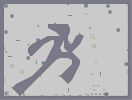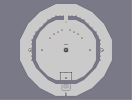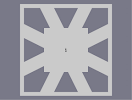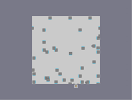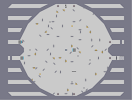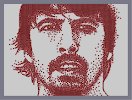the big mount-N #2 Down in One free tiles anyone? thwumpty dumpty 1 minute wonder My Hero

Yeah...

### Ummm,

he reminds of the guy from 'A Series of Unfortunate Events'. But that's pretty good anyway. 4.5

### Lol

Looks like Harry Potter

### Lol

Looks like Harry Potter

### Ooo

One of the few N Arts that looks as good in full-view as it does in the thumbnail! Nice!!

### Teh Suki!

Nice! Better than the other, but only because you didn't only use mines. 5aved.

just wow.

5 lol. arghhh

### it..

looks better if you squint your eyes when looking at it full screen, i think...

### really great

it's one of the best N-arts I've seen!
4.5/5
fav

### i actually

saw suki in that image.....wow.....5/5

### YES!!!

the subject likes my work. better than any rating could compare to. im happy knowing that he likes it lol. although staring at a pic of suki for about 4 hours cant be healthy. >_<

WOWOWOWOW!

### ...

I mean this one:
http://metanet.2.forumer.com/index.php?showtopic=1432&view=findpost&p=24516

### Who's that handsome guy!?

Yeah, that's totally me.
Thanks, wedgie. This totally kicks ass.

Though I would've appreciated a pic in which I didn't look so wierd, like...
http://metanet.2.forumer.com/index.php?showtopic=1432&st=0#
...I can't say I'm not blown away by your work. It's absolutely incredible (and not just because it's me, though we all know that's reason enough).

### haha...

nice one. I'll let Suki know.

### Ignore

The part about the mines. I took them out myself and it looks naff.

### Suki!

I don't like the mines on the lips, I thnk it would have worked much better without, blended in more, and the colour makes him look ill. considering what you're working with though, it's pretty great.

The hair is the best part.

Watching it draw itself in NED is really satisfying.

### also

its really hard to do this and get the shading to look right just using gausses lol.
dragon moons dragons are awesome but im different in style. as his are mythical creatures whereas i am trying to get the best likeness to the subject. in this case suki lol. although yeah its not great. :/

### the dragons by dragon_moon are better!!

But this hero is, of course, 5\5

### WOW!!!

amazing again! 5/5 really really really great!

5/5 njas

### lol

although the comparison of the two may not look that good. that picture is still woth being seen.

### Whoops I meant here:

http://metanet.2.forumer.com/index.php?showtopic=1432&st=480&#entry129537

### LOL!

well black vs blonde hair for a start. :D

### O...

I just got it. Its funny especially when you look here:
http://metanet.2.forumer.com/index.php?showtopic=1432&st=480

### Tis Suki!

For a minute, I thought it was Wedgie himself, but than I realized that it wasn't. Major differences at a second glance.

Still a 5 though.....

### it looked like Suki

dont feel like rating, maybe later

### lol

yeah you may appreciate the likeness and subject more if you knew who it was. NIGHTMARE knows lol. its not brilliant but still worth a map lol.
dogdog234: im not an artist by profession but i have studied art for most of my school life lol.

why?

### its

suki! teh awesum!

### WHOA!

you are so talented with art... are u thinking of becoming an artist?

### lol...

i suggest if anybodies running anything less than an AMD 64 3000+ to not try to load it....unless you really really want to...cuz it doesnt make too much difference in its looks...just enlarge the thumbnail...its close enough

### im with guiseppi....

good job...not perfect, but still excellent...

but hes ugly... i say it should be in top 15 or 20 arts...and that may be a little harsh, i dont know, i guess we will see...

### yeah

i didnt want to add a background because of fear of 'losing' the face in it. it would also kill me with the loading time. this took me about 3-4 hours ish lol. i wasnt going to submit it at first because it didnt look as good on here as it did at first lol. another thing i wasnt expecting is that the ninja is stood legs together here, whereas in edit mode he stands in a running stance, therefore changing the look of the hair lol. the layer ordering has also screwed up here. oh well.

### ARGH

sorry for commenting so much, hmm maybe you can put something in backround... Um it might turn out bad nvm dont ruin it

### I like it

better close up.. lol he's not that ugly... Is this from a book?? Nice artwork dude, again nice artwork... err he's freaking me out now